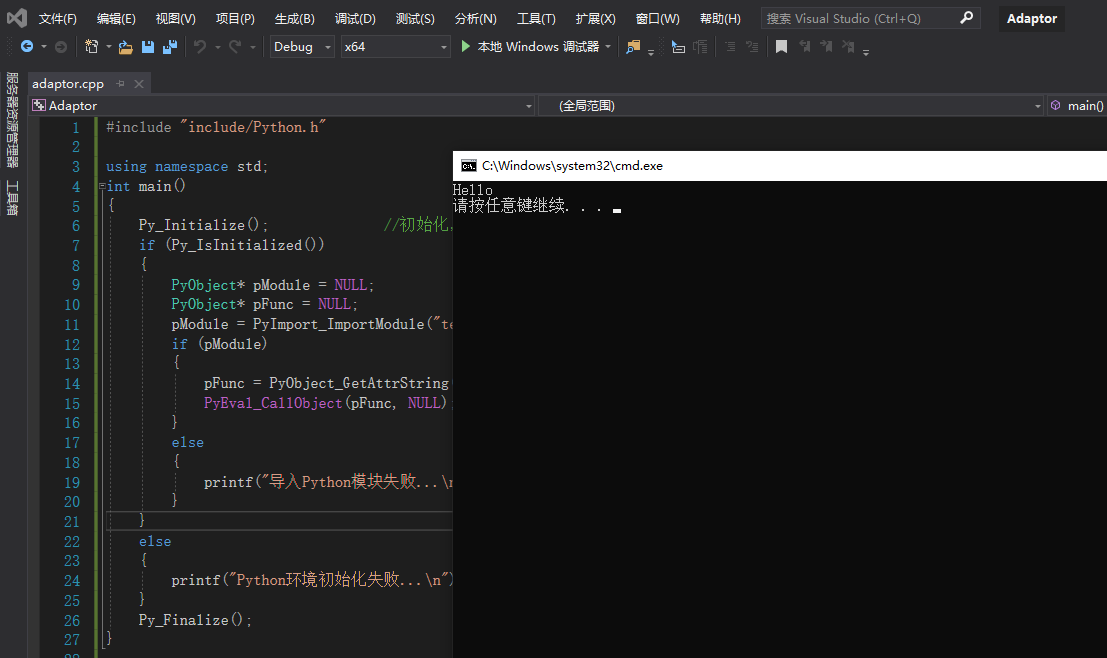# C++ 调用Python脚本

Windows10；

python3.7.4；

visual studio 2019；

libs目录下的python37.lib复制一份为python37_d.lib

def Hello():
print("Hello")

def Add(a,b):
return  a+b

#include <Python.h>
using namespace std;

int main()
{
Py_Initialize();              //初始化，创建一个Python虚拟环境
if (Py_IsInitialized())
{
PyObject* pModule = NULL;
PyObject* pFunc = NULL;
pModule = PyImport_ImportModule("test_python");  //参数为Python脚本的文件名
if (pModule)
{
pFunc = PyObject_GetAttrString(pModule, "Hello");   //获取函数
PyEval_CallObject(pFunc, NULL);           //执行函数
}
else
{
printf("导入Python模块失败...\n");
}
}
else
{
printf("Python环境初始化失败...\n");
}
Py_Finalize();
}ok，成功调用python脚本。

#include<Python.h> //添加python的声明

using namespace std;

int main()
{
Py_Initialize(); //1、初始化python接口

//初始化使用的变量
PyObject* pModule = NULL;
PyObject* pFunc = NULL;
PyObject* pName = NULL;

//2、初始化python系统文件路径，保证可以访问到 .py文件
PyRun_SimpleString("import sys");
PyRun_SimpleString("sys.path.append('./')");

//3、调用python文件名。当前的测试python文件名是test.py。在使用这个函数的时候，只需要写文件的名称就可以了。不用写后缀。
pModule = PyImport_ImportModule("test");

//4、调用函数
pFunc = PyObject_GetAttrString(pModule, "AdditionFc");

//5、给python传参数
PyObject* pArgs = PyTuple_New(2);//函数调用的参数传递均是以元组的形式打包的,2表示参数个数。如果AdditionFc中只有一个参数时，写1就可以了。这里只先介绍函数必须有参数存在的情况。

PyTuple_SetItem(pArgs, 0, Py_BuildValue("i", 2)); //0：表示序号。第一个参数。
PyTuple_SetItem(pArgs, 1, Py_BuildValue("i", 4)); //1：也表示序号。第二个参数。i：表示传入的参数类型是int类型。

//6、使用C++的python接口调用该函数
PyObject* pReturn = PyEval_CallObject(pFunc, pArgs);

//7、接收python计算好的返回值
int nResult;
PyArg_Parse(pReturn, "i", &nResult);//i表示转换成int型变量。在这里，最需要注意的是：PyArg_Parse的最后一个参数，必须加上“&”符号。

//8、结束python接口初始化
Py_Finalize();
}


posted @ 2019-09-05 16:26  Ariel_一只猫的旅行  阅读(9613)  评论(0编辑  收藏